International
Tables for
Crystallography
Volume A
Space-group symmetry
Edited by M. I. Aroyo

International Tables for Crystallography (2015). Vol. A, ch. 1.4, pp. 61-62

## Section 1.4.4.1. Crystallographic orbits

B. Souvignierd

#### 1.4.4.1. Crystallographic orbits

| top | pdf |

Since the operations of a space group provide symmetries of a crystal pattern, two points X and Y that are mapped onto each other by a space-group operation are regarded as being geometrically equivalent. Starting from a point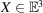, infinitely many points Y equivalent to X are obtained by applying all space-group operations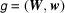to X: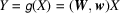=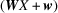.

#### Definition

For a space groupacting on the three-dimensional space, the (infinite) set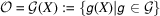is called the orbit of X under.

The orbit of X is the smallest subset ofthat contains X and is closed under the action of. It is also called a crystallographic orbit.

Every point in direct spacebelongs to precisely one orbit underand thus the orbits ofpartition the direct space into disjoint subsets. It is clear that an orbit is completely determined by its points in the unit cell, since translating the unit cell by the translation subgroupofentirely covers.

It may happen that two different symmetry operations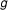andinmap X to the same point. Since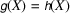implies that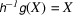, the point X is fixed by the nontrivial operation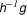in.

#### Definition

The subgroup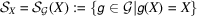of symmetry operations fromthat fix X is called the site-symmetry group of X in.

Since translations, glide reflections and screw rotations fix no point in, a site-symmetry group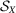never contains operations of these types and thus consists only of reflections, rotations, inversions and rotoinversions. Because of the absence of translations,contains at most one operation from a coset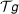relative to the translation subgroupof, since otherwise the quotient of two such operations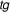and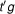would be the non-trivial translation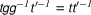(see Chapter 1.3for a discussion of coset decompositions). In particular, the operations inall have different linear parts and because these linear parts form a subgroup of the point groupof, the order of the site-symmetry groupis a divisor of the order of the point group of.

The site-symmetry group of a point X is thus a finite subgroup of the space group, a subgroup which is isomorphic to a subgroup of the point groupof.

#### Example

For a space groupof type, the site-symmetry group of the origin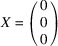is clearly generated by the inversion in the origin: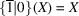. On the other hand, the point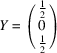is fixed by the inversion in Y, i.e.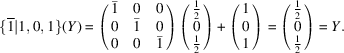The symmetry operation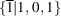also belongs toand generates the site-symmetry group of Y. The site-symmetry groups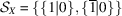of X and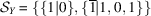of Y are thus different subgroups of order 2 ofwhich are isomorphic to the point group of(which is generated by).

The order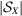of the site-symmetry groupis closely related to the number of points in the orbit of X that lie in the unit cell. An application of the orbit–stabilizer theorem (see Section 1.1.7) yields the crucial observation that each point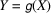in the orbit of X underis obtained preciselytimes as an orbit point: for each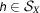one has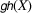=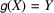and conversely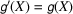implies that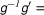and thus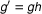for an operationin.

Assuming first that we are dealing with a space groupdescribed by a primitive lattice, each coset ofrelative to the translation subgroupcontains precisely one operationsuch that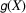lies in the primitive unit cell. Since the number of cosets equals the order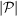of the point groupofand since each orbit point is obtainedtimes, it follows that the number of orbit points in the unit cell is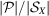.

If we deal with a space group with a centred unit cell, the above result has to be modified slightly. If there are k − 1 centring vectors, the lattice spanned by the conventional basis is a sublattice of index k in the full translation lattice. The conventional cell therefore is built up from k primitive unit cells (spanned by a primitive lattice basis) and thus in particular contains k times as many points as the primitive cell (see Chapter 1.3for a detailed discussion of conventional and primitive bases and cells).

#### Proposition

Letbe a space group with point groupand letbe the site-symmetry group of a point X in. Then the number of orbit points of the orbit of X which lie in a conventional cell foris equal to the product, where k is the volume of the conventional cell divided by the volume of a primitive unit cell.